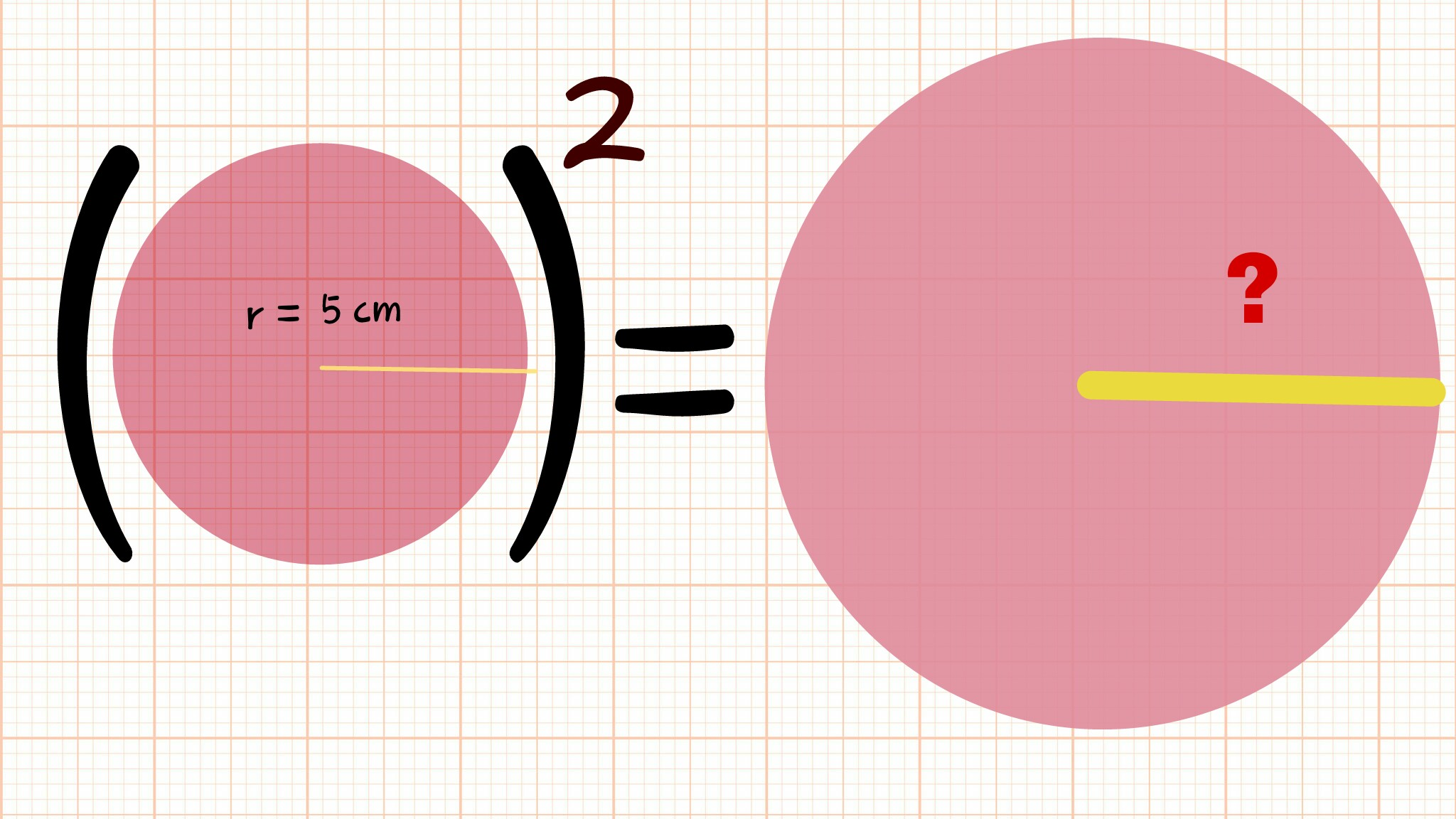# A square of circle

Geometry Level 2There are two circles of different sizes, the smaller circle has a radius of 5. The numerical value of the square of the area of the smaller circle is equal to the numerical value of the area of the larger circle. Find the radius of the larger circle.